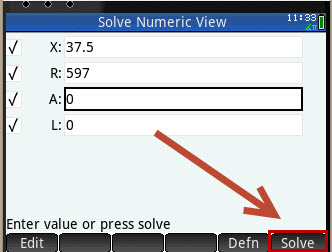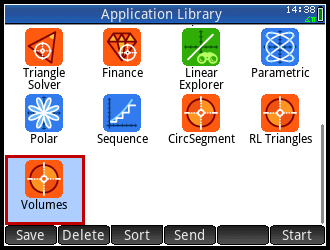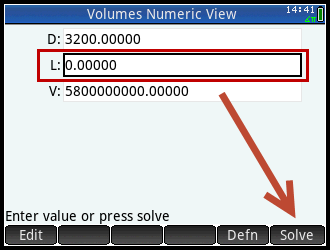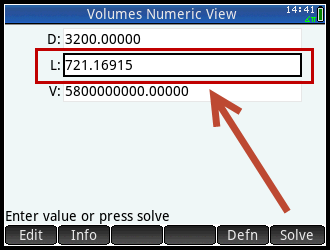cancel
Showing results for
Did you mean:

### Note on archived topics.

This topic has been archived. Information and links in this thread may no longer be available or relevant.
If you have a question create a new topic by clicking here and select the appropriate board.
Highlighted
Level 3
41 31 2 1
Message 1 of 20
3,617
Flag PostHP Recommended

Solved!

# HP 50G Solver equation transfer to HP Prime

Hi,

My next question relates to my commonly used work equations that I have stored on my HP 50G.

I use these to solve for an unknown variable - and they are used quite a lot.

Is there anyway to transfer these to the HP Prime?

If yes, how? How do you store them and where?

On the HP 50G, for instance, they are in their specific directories - for example

Home

|- Circsect

|- Areas

|- Circring.............0.7854x(O^2-I^2)-A=0  <---to find either O; I or A

|- Circular

|- Rect

|- Sq

|- Volumes

|- Rect

|- Cyl......................0.78540xD^2xL-V=0 <- to find either D; L or V

|- HollCyl

|- Cone

and so on - specific to what we work on....

I do not know where to start with this one. So any help would be appreciated ina simplified format.

Regards,

FG

Tags (3)
19 REPLIES 19
Level 3
41 31 2 1
Message 2 of 20
3,534
Flag PostHP Recommended

Solved!

## HP 50G Solver equation transfer to HP Prime

OK......obviously this topic is either too hard to do or no one knows how to do it - or maybe it can't be achieved.

Therefore, lets forget any transfer from the 50G!

Will I able to reproduce the various formula shown below on the HP Prime, store the formula and recall the formula to Solve individually?

Such as I input the following formulas, so I am able to solve for any unknown variable.

Example #1

Input:

0.78540xD^2xL-V=0 <- to find either D; L or V

How and where do I then store this "CylVol" on the HP Prime?

How do i recall this formula to solve at another time?

Example #2

Input:

0.7854x(O^2-I^2)-A=0 <---to find either O; I or A

How and where do I then store this "RingArea" on the HP Prime?

How do I recall this formula to solve at another time?

And so on....

I do not know where to start with this one. So any help would be appreciated in a simplified format.

Regards,

FG

Level 1
9 9 0 0
Message 3 of 20
3,515
Flag PostHP Recommended

Solved!

## HP 50G Solver equation transfer to HP Prime

Hi FG,
check out the user guide starting at page 107 to about page 110. You will be using the SOLVE APP. If you still have questions after that please post again for more help.
Level 3
41 31 2 1
Message 4 of 20
3,467
Flag PostHP Recommended

Solved!

## HP 50G Solver equation transfer to HP Prime

Hi Jimsz,

Before I start making up apps, copying apps and so on I thought I would try one of the calculation sets that I use in the HP 50G as a test for functionality first in the HP Prime.

OK....so using the "Solver App" (is this the correct application?) for my Circular Segment "Circseg" formulas.

For this example, I usually know two variables, so I input what is known...

Angle "X" = 37.5

So I enter in the necessary formulas (that I already use stored in my 50G) as shown:On then picking the Numeric key, it then takes me to the variable information Input boxes....so far so good.

I input for "X" and "R" (as indicated above):However, after clicking on Solve, it fails!I even tried this with the formulas as =A; =R; =L, etc....such as

0.00873*X*R^2=A

((57.296*L)/X)=R

and so on, but still failed.

So either I have the overall concept of the solver totally wrong, or I am using the wrong format for doing this....

Any (understandable and simple) suggestions or advice would be very welcome...

Regards,

FG

Level 1
9 9 0 0
Message 5 of 20
3,452
Flag PostHP Recommended

Solved!

## HP 50G Solver equation transfer to HP Prime

Hi FG,

I keyed in your equations and ran them. I solved them one at a time.

Uncheck all except for the first one (E1) and you get a value for A.

Uncheck E1 and check E2. Solve to get a value for L. Uncheck E2 and check E3 and solve for L again.

I got a value of 390.734082659.

Uncheck E3 and check E4. Solve for l again. Here I found a problem! L= 390.661875 ?

Is the real constant 0.01745 correct?

Hope this helps some.

jim

Level 3
41 31 2 1
Message 6 of 20
3,448
Flag PostHP Recommended

Solved!

## HP 50G Solver equation transfer to HP Prime

Hi,

Thanks, yes - single equation works good in general.

However, as you state I am getting some strange answers sometimes, when checked against answers on the 50G.

I just did an App based on the Solve App - Called "Volumes" and singularly can solve for an unknown variable OK, using the formula individually.

However, this brings me to my next little or major issue.....

This is the App - copied and saved:On picking the App, I have the following formulas listed (as per 50G)...For this example I have picked E4: which is my equation for the Cylinder Volume.

I can then go to Numeric.....Input my KNOWN Varaiables, in this case the maximum volume (mm3) and the required diameter (mm) of the cylinder, so then I can Solve for the 'minimum height' of my cylinder....Which is solved as 721.16915 (mm), I would then normally round this value to a number such as 725 or 730 (mm).

So far so good and the fuctions worked....

However, my big problem with this and any of the Apps produced, is picking quickly the correct formula I require....!

In the 50G, under 'Volumes', each of the formulas are covered by a small descriptor such as Sphere; Rect; Cube, Cylinder; HollCyl, etc.

So by picking this title I got the rquired formula correctly and quickly!

Currently (because I have only just done the app, so remember what is where), I have is this...

E1: = Sphere

E2: = SQEnd

E3: = Rect

E4: = Cyl

E5: = Cube

E6: = HollCyl

E7: = Cone....

In its present state, how can I either add a description to the labels E1:, E2:, E3:, etc. or change these into meaningful titles, as above?

Cheers

FG

Level 3
41 31 2 1
Message 7 of 20
3,447
Flag PostHP Recommended

Solved!

## HP 50G Solver equation transfer to HP Prime

Hmmmm......I get L= 390.661875 also!

Don't know what to say, as the formulas are taken from Machinery's Handbook - Edition 26.....so as far as I know all the values stated are correct as written.

I will have to recheck, now that you have brought it to my attention....

Cheers,

FG
Level 1
9 9 0 0
Message 8 of 20
3,430
Flag PostHP Recommended

Solved!

## HP 50G Solver equation transfer to HP Prime

Hi FG,

Glad you got some of the equations to work.

What I do to help me remember is to create a Note with the same name as the Icon used and put a short explanation of each E. for example :

E1 calculates volume

E2 calculates this

E3 calculates that

etc.

So before or during I can do SHIFT NOTES and choose the name I gave to the APP and see what all that stuff does. Works pretty slick for me.

Hope this helps.

Jim

Level 3
41 31 2 1
Message 9 of 20
3,423
Flag PostHP Recommended

Solved!

## HP 50G Solver equation transfer to HP Prime

Hi Jim,

I finally got all equations to work...and refined some of the formula, to be their original values.....such (Pi/180)......or (360/Pi), etc....that did it.

Also, the other equations I had problems with were because the anglular values were set to radians and not degrees. Once I set to degrees all was good! I wonder why default isn't degrees...?

Regarding your last post (and thank you for your guidance by the way) I am not sure what you meant by the following....

"What I do to help me remember is to create a Note with the same name as the Icon used and put a short explanation of each E. for example :

E1 calculates Area

E3 calculates Angle

E4 calculates Arclength

E5 calculates Chord

etc.

So before or during I can do SHIFT NOTES and choose the name I gave to the APP and see what all that stuff does. Works pretty slick for me."

Could you please explain this a bit better or what to do - maybe a step by step example...?

Cheers

FG

Level 1
5 5 1 3
Message 10 of 20
3,419
Flag PostHP Recommended

Solved!

## HP 50G Solver equation transfer to HP Prime

Hi,

Maybe Jim should have said Shift 0. That is the shortcut to the Notes app on the calculator. I.e. Shift Notes would be typed "Shift" button "0" (Zero) button.

Cheer

### Note on archived topics.

This topic has been archived. Information and links in this thread may no longer be available or relevant.
If you have a question create a new topic by clicking here and select the appropriate board.
† The opinions expressed above are the personal opinions of the authors, not of HP. By using this site, you accept the Terms of Use and Rules of Participation